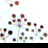# Kernal Density results - units???

2525
7
08-19-2013 09:49 AMNew Contributor III
Hi all,

I am running a Kernal Density analysis on a point layer (sewage overflow locations).  The coordinate system I am using is CA State Plane NAD 83 FEET.  My inputs include:

Point features: OverflowLocs
Population field: NONE (this means by default that each point will be counted once)
Output Raster: OverflowDens
Output cell size: 50 (feet)
Search radius 880 (feet) = 1/6 mile which suits my needs for neighborhood/block level data
Area units: Square Miles

Using these parameters, my result looks great, but I don't understand the units of the output values:
lowest (coolest) value range: 0 - 6.6259
highest (hottest) value range 205.4043 - 336.5979

The ArcGIS Help describes the output as "magnitude per unit area".  To me, this means that the highest value then reads as 336.5979/1sq mi.  This is considering that my input Area Units were Square Miles, so I'm assuming that my "unit area" is 1 square mile.  Correct?

Further, what are the units (or at least a generic definition) for "magnitude" in this case?  Is it the density of the points (ie. points per sq mile? Or is it points per search radius (880 feet), or some other units?

Christi
Tags (2)
1 Solution

Accepted SolutionsbyEsri Regular Contributor
It's just 336.5979 points (sewage overflow locations)/ sq mile.  If you haven't read the blog below, I think it may be helpful.

How should I intrepret the output of density tools?

In particular, you may be interested in converting density into expected count.

Best,
Eric
7 RepliesbyEsri Regular Contributor
Did you check out How Kernel Density works for point features?

Best,
EricNew Contributor III
Yes, thanks.

I just need confirmation/clarification about the units in the result values.

That site says that Kernal Density calculates "the density of point features around each output raster cell".  So my output raster cell size is 50 feet, but my Area units are in Square Miles.

The ArcGIS Help says that the tool calculates the "magnitude per unit area".

If my output value is 336.5979...what are the units?byEsri Regular Contributor
If an area unit is selected, the calculated density for the cell is  multiplied by the appropriate factor before it is written to the output  raster.

To see the effect of going to sq miles, set the area unit to SQUARE_MAP_UNITS (your original unit feet).

Best,
EricNew Contributor III
Yep!

Thats exactly what I did - so glad I did it correctly.

So in my map legend, when I have a color ramp from red to blue (high density to low density), I will put the highest value at the top (next to the red).  This highest value is 336.5979.   So what are the units of this number?  336.5979 ?/sq mile?  (see attachment)

Sorry, I just know that my client is going to ask and I need to be sure.byMVP Esteemed Contributor
Sorry, I just know that my client is going to ask and I need to be sure.

Given the ease of getting this wrong,  I double check by doing the calculation "by hand" for a cell or two. This isn't that hard to estimate using the measure tool in ArcMap.

As a longtime GIS colleague and friend likes to quote: "Trust, but verify."byEsri Regular Contributor
It's just 336.5979 points (sewage overflow locations)/ sq mile.  If you haven't read the blog below, I think it may be helpful.

How should I intrepret the output of density tools?

In particular, you may be interested in converting density into expected count.

Best,
EricNew Contributor III
It's just 336.5979 points (sewage overflow locations)/ sq mile.  If you haven't read the blog below, I think it may be helpful.

How should I intrepret the output of density tools?

In particular, you may be interested in converting density into expected count.

Best,
Eric

Thank you so much!